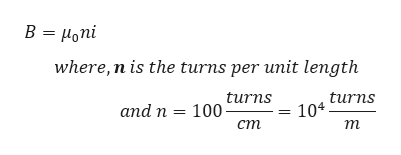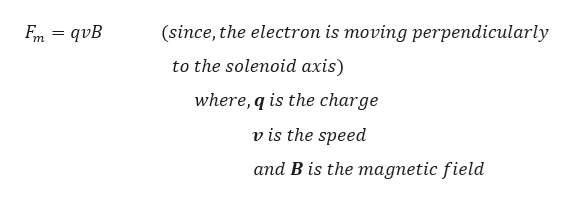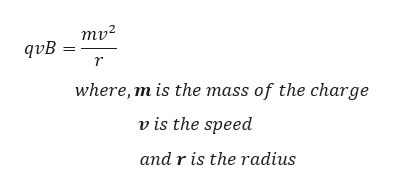A long solenoid has 100 turns/cm and carries current i. An electron moves within the solenoid in a circle of radius 3.24 cm perpendicular to the solenoid axis. The speed of the electron is 0.0469c (c = speed of light, equal to 2.998 × 108 m/s). Find the current i in the solenoid.

Question

A long solenoid has 100 turns/cm and carries current i. An electron moves within the solenoid in a circle of radius 3.24 cm perpendicular to the solenoid axis. The speed of the electron is 0.0469c (c = speed of light, equal to 2.998 × 108 m/s). Find the current i in the solenoid.

Step 1

Magnetic field in the solenoid is given by :help_outlineImage TranscriptioncloseB Honi _ where, n is the turns per unit length turns 104. т and n = 100 urns ст fullscreen
Step 2

We know that, force on the moving electron in a magnetic field will be:help_outlineImage Transcriptionclose(since, the electron is moving perpendicularly En = qvB to the solenoid axis) where, q is the charge v is the speed and B is the magnetic field fullscreen
Step 3

Now, the magnetic force will be equal to the centripetal f...help_outlineImage Transcriptionclosemv2 qvB r where, m is the mass of the charge v is the speed and r is the radius fullscreen

Want to see the full answer?

See Solution

Want to see this answer and more?

Our solutions are written by experts, many with advanced degrees, and available 24/7

See Solution
Tagged in

Magnetic Effects of Current